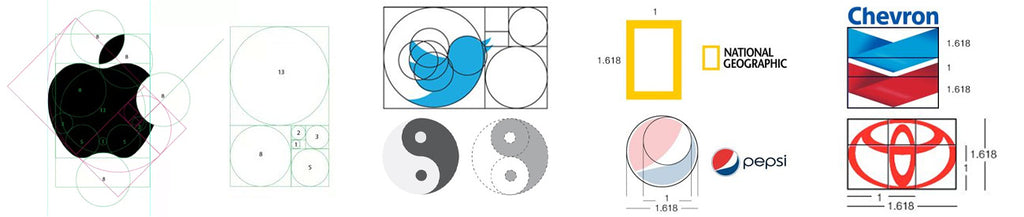# Episode 2: The Golden Ratio

### What is the Golden Ratio?

In mathematics, two quantities are in the Golden Ratio if their ratio is the same as the ratio of their sum to the larger of the two quantities:where the Greek letter phi (Φ or φ) represents the Golden Ratio.

Maybe a simpler way look is if we divide a line in 2 parts, so that the whole length divided by the long part is also equal to the long part divided by the short part, we find the Golden Ratio.It is an irrational number like pi and e, meaning that its terms go on forever after the decimal point without repeating.
It's (truncated) value is 1.61803398874989484821...

### What is its origin?

The origin of this number can be traced back to Euclid, who then called it the “extreme and mean ratio” in the Elements. Euclid’s Elements (c. 300 BCE) is the oldest enduring large-scale deductive treatment of mathematics, which guided mathematicians, philosophers, and scientists well into the 19th century.

But the first known calculation of the Golden Ratio, as a decimal, was given in a letter written by Michael Mästlin in 1597, at the University of Tübingen, to his former student Johannes Kepler. Michael Mästlin calculated the Golden Ratio as 1.6180340 (it's value is 1.61803398874989484821... Not a bad approximation, considering it was 1597).

### Why is the Golden Ratio the 2nd most famous irrational number?

Pi (π) is unequivocally the most famous irrational number, but the Golden Ration (Φ) is arguably considered as the 2nd most famous one. Probably because over the centuries, the idea that it represents perfect beauty or is uniquely found throughout nature has been perpetuated:
Italian Renaissance mathematician, Luca Pacioli, wrote a book called "De Divina Proportione" ("The Divine Proportion") in 1509 that discussed and popularized the Golden Ratio.
Novelist Dan Brown, in his bestselling book "The Da Vinci Code", included a long passage where the main character, Robert Langdon, discusses how Φ represents the ideal of beauty.
With some approximation, the Golden Ration has also been used in logos we all know, such as Pepsi, Twitter, Apple, National Geographic, Chevron, Toyota, and more.### How does the Golden Ratio apply in Nature?

We covered some of it in our Episode 1, The Fibonacci sequence. We can observe the Golden Ratio in over a dozen of examples in nature:

1. Mammals (Dolphins, Ram's horns...)
2. Insects (Ants' body sections)
3. DNA molecules (the ratio between its length and width)
4. Faces
5. Fingers (each phalange compared to the next)
6. Flowers (Sunflower, Daisy, Passion flower...)
7. Fruits and their seeds arrangements (Apple, Star fruit, Okra...)
8. Vegetables (Romanesco Broccoli, Artichoke...)
9. Hurricanes (in a Golden Spiral shape)
10. Reproductive dynamics ()
11. Shells (Nautilus)
12. Spiral Galaxies (its arms can be traced as Golden Spirals)
13. Tree branches (how they split in a Fibonacci sequence)

Some might call-out approximations to the Golden Ratio, for example when looking at the DNA molecule (The DNA molecule measures 34 angstroms long by 21 angstroms wide for each full cycle of its double helix spiral. These numbers, 34 and 21, are numbers in the Fibonacci series, and their ratio 1.6190476 closely approximates Φ, 1.6180339.). But both numbers are indeed consecutive numbers of the Fibonacci sequence.

Sceptic or not, it is interesting to see how patterns emerge from Nature or how it keeps on amazing us.

“The best classroom and the richest classroom is roofed only by the sky” [Margaret McMillan]